## A Bit More on Debt and Growth

Here is a bit more analysis of the data set used by Reinhart and Rogoff (R-R) and by Herndon Ash and Pollin (HAP) in their critique, and in particular the stata data set RR-processed.dta with data on public debt to GDP ratios and real GDP growth in 20 developed countries since 1946.  This post is a minor addition to my earlier post and, especially adds little not shown by  Arindrajit Dube.

In general macroeconomists have a problem that we non experimental data so we observe correlation but we want to know about causation.  We don’t know what to do about this problem, so we almost all do the simplest thing which is look at timing — the cause comes before the effect. This is what Dube did showing a much stronger relationship between the public debt to gdp ratio and real GDP growth in the preceding 3 years than between the ratio and growth in the following three years.  Also the non parametric estimate suggest a much stronger relationship between higher debt and lower subsequent growth for low levels of debt (up to 30%  of GDP) than for higher levels — the opposite of what policy makers guessed given the original Reinhart and Rogoff analysis which really didn’t address that issue.

I redo Dube with parametric regressions which have the nice feature that there are standard statistical tests of null hypotheses concerning parametric point estimates (that’s fancy talk for STATA gives me t-statistics).  The very first standard way to look at causality using post hoc ergo propter hoc is caused a Grander causality test. It is just a regression of one variable on lagged values of the explanatory variable and lagged values of the dependent variable.  This is definitely the first thing macroeconomists do does if he she or they wonder about the direction of causality.

Recall the basic regression which notes that a debt to GDP ratio 1% higher is associated with a real GDP growth rate which is 0.02% lower (this is in fact a big deal).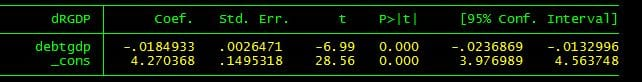dRGDP is the rate of growth of real GDP in the country, debtgdp is the ratio of public debt to gdp.

Now  l1drgdp is the rate of real GDP growth lagged one year.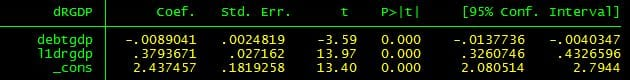Including that variable appears to show a striking decline in the coefficient on the debt to GDP ratio,  but really one should calculate the long term effect of a permanent increase in the debt ratio using

(1-0.3793671)(new steady state growth rate) = constant -0.089041(debt to GDP ratio) so the estimated long term effect is about -0.015 not much smaller than the simple coefficient.

The t-statistic is calculated assuming that the disturbance to growth is independent across countries (no global recessions or oil shocks) and that all structure within a country is captured by the simple lagged term.  STATA is very willing to recalculate standard errors assuming, for example, that disturbances in the same year may be correlated.
. reg dRGDP debtgdp l1drgdp, cluster(Year)

gives a t-statistic on the debt to GDP ratio of -2.45 (the point estimates are exactly the same the cluster option just calculates more robust estimates of the standard errors).  No big change.

Now I can get the t-statistic to be insignificant (you can always do this).  I do this by definining a new lagged variable al3drgdp which is the average of the growth rate of real GDP lagged one, two, and three years.   When I toss that in the regression and calculate standard errors allowing correlation in the same year across countries I get the t-statistic insignificant.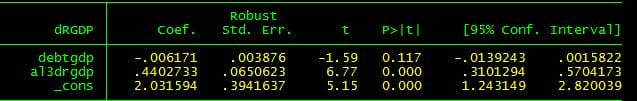There is nothing wrong with this regression, except that I fiddled till I got the absolute value of the t-statistic under one (the added variable is due to Dube so there is that).    The point estimate of the effect on growth isn’t much lower.  the long run guess is a bit below 0.01 so slightly less than half the estimate based on the simple regression.

There is nothing much to see here.  Neither strong evidence that the original estimate is due to reverse causation nor strong evidence that high debt causes low growth.

In contrast, the hypothesis that low growth causes a high debt to gdp ratio is not just obviously true but also strongly supported by the data.  Here l1debtgdp is the lagged ratio of public debt to GDP.
. reg debtgdp l1drgdp l1debtgdp, cluster(Year)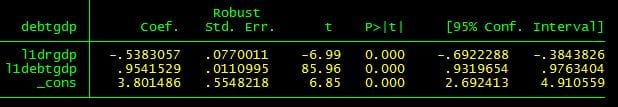Note the t-statistic is robust to correlation across countries within a year.  Similarly, if STATA allows correlation of disturbances for the same country at different years (but not across countries within a year) the t-statistic is still huge being -6.21.

This shows that you just can’t interpret the simple coefficient as a measurement of the effect of debt on growth.  But we knew that (R-R never denied that there was some reverse causation).

Now for something slightly different.  How about non linearity ?  A lot of interest in R-R was due to the unreasonable interpretation that their analysis showed a threshold near a debt to gdp ratio of 90%.   I am going to go back to regressing real GDP growth on lagged real GDP growth and data on the debt ratio but cut the data on the debt ratio to debtgdpto90 which is rounded down to 90 if the ratio is over 90%

gen debtgdpto90 = debtgdp + (90-debtgdp)*(debtgdp>90)

and gen debtgdpmin30 = debtgdp-debtgdpto30 which is zero if the ratio is less than 90 and the ratio minus 90 if the ratio is greater than 90

The results correspond to the pattern reported by Dube (as they must) the point estimate of the effect of debt over 90% is lower and only borderline statistically significantly different from zero.

reg dRGDP debtgdpto90 debtgdpmin90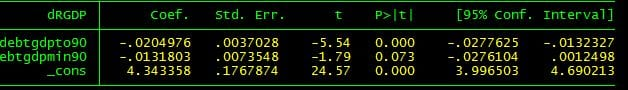Now how about both considering non-linearity and including a lagged dependent variable for Granger “causality”

reg dRGDP debtgdpto90 debtgdpmin90 l1drgdp

There is no evidence at all that increasing the debt to GDP ratio above 9o% has a negative causal effect on real GDP growth.  In my heart,  I am sure that debt still does have such an effect even if it is over 90%  but there is just no evidence for this effect in R-R’s famous data set which was interpreted as including strong evidence that the effect is very large.

Finally out of respect for Dube, I consider debt ratios rounded down to 30% debtgdpto30 and above 30% debtgdpmin30

. reg dRGDP debtgdpto30 debtgdpmin30

As we know from Dube 2013, debt appears to make a huge difference up till a ratio of 30% then much less.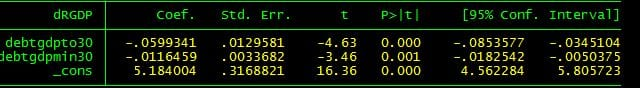Finally (really finally the end at last) the null of no Granger “causality” is not rejected for ratios above 3o% .  The evidence is that having very low debt ratios has a good effect on growth, but above that low level, there isn’t statistically signficant evidence of a causal effect.

reg dRGDP debtgdpto30 debtgdpmin30 l1drgdp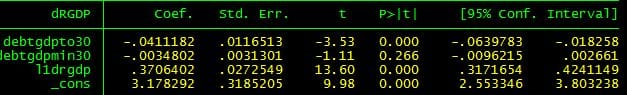here is the do file I used to generate the regressions I just cut and pasted

clear
use C:\rjw\Papers\Peri\RR-processed.dta

quietly tab Country,gen(count)
gen cntry = count1+2*count2+3*count3+4*count4+5*count5+6*count6 + 7*count7 + 8*count8+9*count9+10*count10+11*count11+12*count12+13*count13+14*count14+15*count15+16*count16+17*count17+18*count18+19*count19+20*count20
quietly tab Year, gen(yr)
sort cntry Year

gen debtcat = 1 + floor(debtgdp/30)
replace debtcat = 4 if debtcat>4
gen episode = 1 in 1/1
replace episode = episode[_n-1]+1-(debtcat==debtcat[_n-1])*(cntry==cntry[_n-1]) in 2/1175

gen debtct1 = debtgdp<30
gen debtct2 = (debtgdp<60)*(debtgdp>=30)
gen debtct3 = (debtgdp<90)*(debtgdp>=60)
gen debtct4 = debtgdp>90

gen debtm90 = (debtgdp-90)*(debtgdp>90)
gen l5debtgdp = debtgdp[_n-5] if cntry==cntry[_n-5]

tab debtcat,sum(dRGDP)
tab debtcat,sum(debtgdp)

reg dRGDP debtgdp debtm90

reg dRGDP debtgdp
reg dRGDP debtgdp if l5debtgdp!=.

reg dRGDP debtgdp l5debtgdp if l5debtgdp!=.

reg dRGDP debtgdp l5debtgdp if l5debtgdp!=., cluster(cntry)

reg dRGDP l5debtgdp if l5debtgdp!=.

reg dRGDP l5debtgdp if l5debtgdp!=.,cluster(cntry)

gen l1drgdp = dRGDP[_n-1] if cntry[_n-1]==cntry
gen l2drgdp = dRGDP[_n-2] if cntry[_n-2]==cntry
gen l3drgdp = dRGDP[_n-3] if cntry[_n-3]==cntry
gen al3drgdp = (l1drgdp+l2drgdp+l3drgdp)/3

gen l1debtgdp = debtgdp[_n-1] if cntry[_n-1]==cntry

gen debtgdpto90 = debtgdp + (90-debtgdp)*(debtgdp>90)
gen debtgdpmin90 = debtgdp-debtgdpto90

gen debtgdpto30 = debtgdp + (30-debtgdp)*(debtgdp>30)
gen debtgdpmin30 = debtgdp-debtgdpto30

reg dRGDP l1drgdp l2drgdp l3drgdp

reg dRGDP debtgdp l1drgdp

reg dRGDP debtgdp l1drgdp, cluster(cntry)
reg dRGDP debtgdp l1drgdp, cluster(Year)

reg debtgdp l1drgdp l1debtgdp
reg debtgdp l1drgdp l1debtgdp, cluster(cntry)
reg debtgdp l1drgdp l1debtgdp, cluster(Year)
reg dRGDP l1debtgdp al3drgdp

reg dRGDP l1debtgdp al3drgdp, cluster(cntry)
reg dRGDP l1debtgdp al3drgdp, cluster(Year)

reg dRGDP debtgdpto90 debtgdpmin90
reg dRGDP debtgdpto90 debtgdpmin90 l1drgdp

reg dRGDP debtgdpto30 debtgdpmin30
reg dRGDP debtgdpto30 debtgdpmin30 l1drgdp